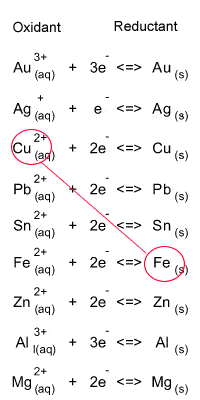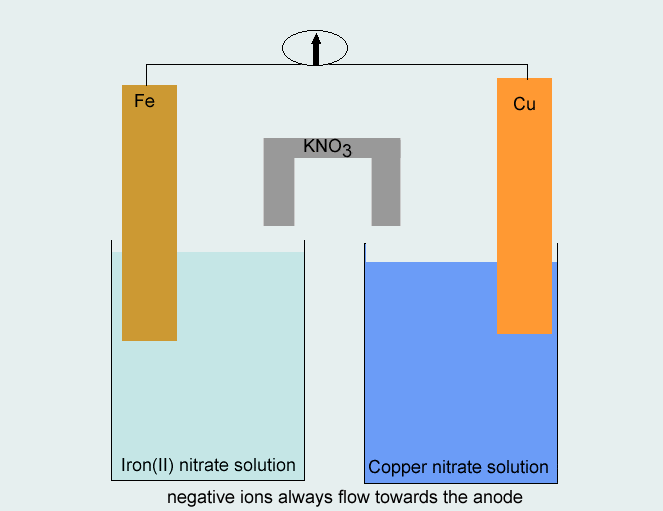Constructing galvanic cells The following pair of half cells are combined to form an electrochemical cell. Fe2+(aq)/ Fe(s) and Cu2+(aq)/ Cu(s) Draw a diagram of the electrochemical cell Indicate the following - direction of electron flow in the external circuit. - direction of negative ions in the salt bridge. - the anode - the cathode - the half equations at each electrode Step 1 Identify the oxidant and reductant from the electrochemical series, as shown on the right. Oxidant = Fe Reductant = Cu2+ Step 2 Write the two half reactions Cu2+(aq) + 2e- <=> Cu(s) ----- reduction Fe(2) <=> Fe2+(aq) + 2e- ----- oxidation Step 3 Use the metals from each half equation as the electrodes in constructing the half cells. A salt solution containing the appropriate ions (Cu2+and Fe2+) should be used in each half cell. The direction of electron flow is from the half cell where oxidation takes place to the half cell where reduction takes place, whereas negative ions move in the opposite direction in the salt bridge.The following pair of half cells are combined to form an electrochemical cell. Ag+(aq)/ Ag(s) and Cu2+(aq)/ Cu(s) Draw a diagram of the electrochemical cell Indicate the following - direction of electron flow in the external circuit. anode to cathode cathode to anode - direction of negative ions in the salt bridge. anode to cathode cathode to anode - the anode - the cathode - the half equations at each electrode - what happens to the mass of the anode? increases decreases stays the same - what happens to the mass of the cathode? increases decreases stays the same Solution Past exam question involving galvanic cells Continue with some more exercises